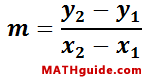Classifying Triangles Using Slope and Distance
Coordinate Geometry Lesson | MATHguide homepage Updated March 20th, 2018

Given:Triangle A(-4, 6), B(0, 9), C(-3, 3)Problem:Determine the slopes of the sides and the lengths of the sides to find characteristics of triangle ABC.

Slopes of Sides
 mAB =mBC =mAC =Lengths of Sides
 dAB =
 dBC =
 dAC =

Characteristics: